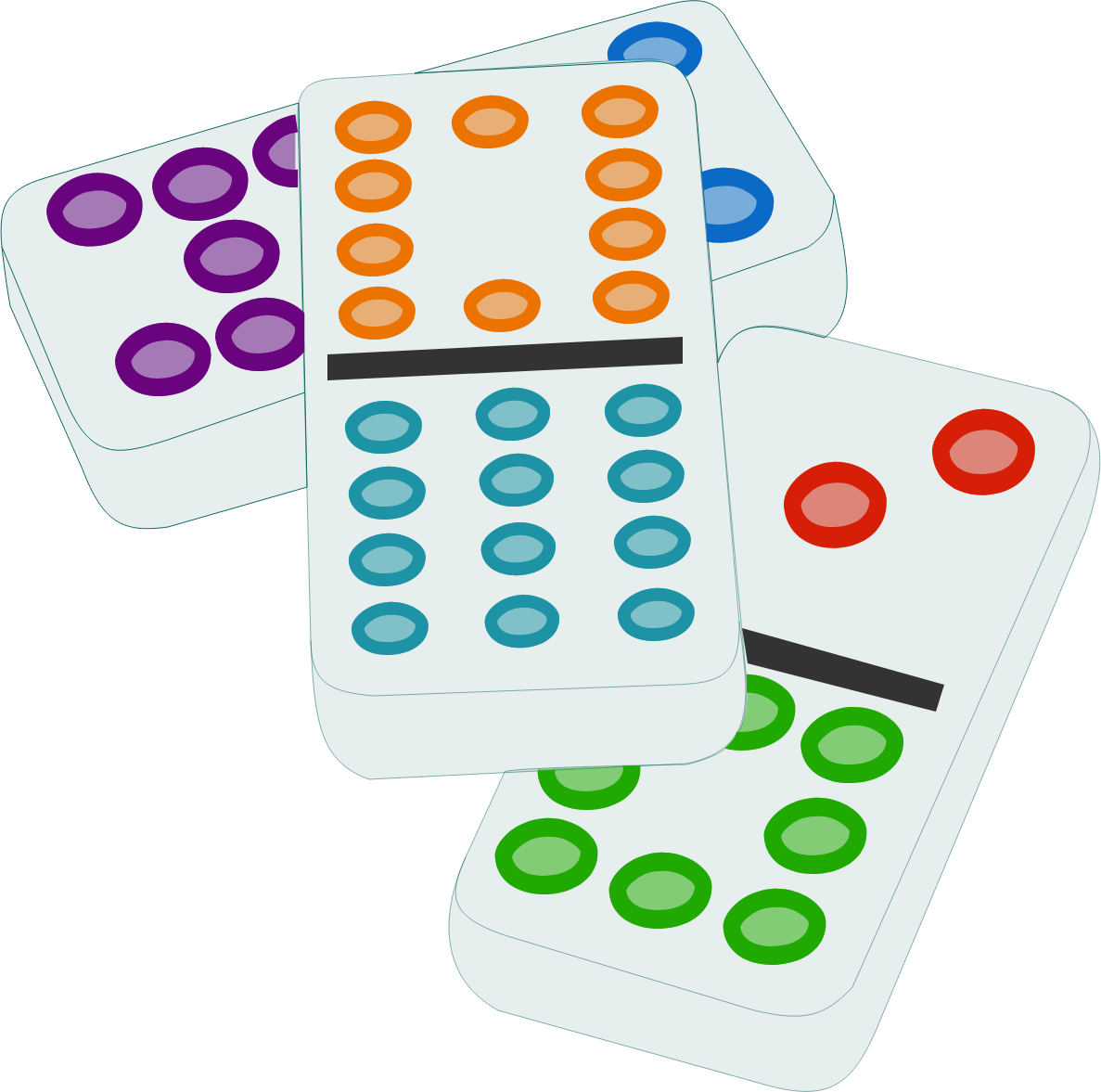# Domino Sums Take 2

Probability Level 2A single domino is a rectangular tile divided into two square ends marked with spots. A domino set of order $n$ consists of all the domino tile combinations with spot counts from $0$ to $n$ inclusive.

$T(n)$ is the sum of the totals of the two spot counts on each tile in the domino set of order $n$, and $P(n)$ is the sum of the products of the two spot counts on each tile in the domino set of order $n$.

For example, a domino set of order $2$ would consist of the domino tiles with numbers $(0, 0)$, $(0, 1)$, $(0, 2)$, $(1, 1)$, $(1, 2)$, $(2, 2)$, such that \begin{aligned} T(2) &= (0 + 0) + (0 + 1) + (0 + 2) + (1 + 1) + (1 + 2) + (2 + 2) \\&= 12\\\\ P(2) &= (0 \cdot 0) + (0 \cdot 1) + (0 \cdot 2) + (1 \cdot 1) + (1 \cdot 2) + (2 \cdot 2) \\&= 7. \end{aligned} What is $\lim_{n\to\infty}} \frac{n T(n)}{P(n)}$

×